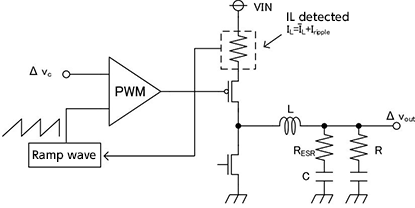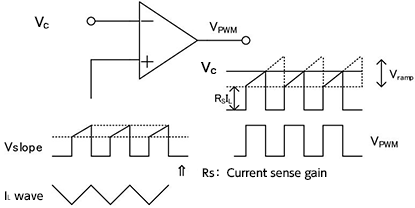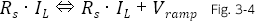Technical Information Site of Power Supply Design

2017.01.12 Transfer Function

# Slope Transfer Functions: Consideration of Current Mode

DC/DC Converters: Sharing of Transfer Functions among Control Systems

In succession to the voltage mode discussed last time, we consider the second type of slope transfer functions, which are current mode transfer functions.Fig. 3

Consideration of current mode

Current-mode control in a DC/DC converter is, together with voltage mode, a basic technique. In the voltage mode, the output voltage Vc of an error amplifier is compared with a fixed slope wave (triangular wave/ramp wave) to determine the PWM signal duty cycle.

On the other hand, in the current mode, control is executed using a slope waveform resulting by adding a fixed ramp wave to the current sense gain (Rs)×coil (inductor) current (IL).

Current-mode slope waveform = fixed ramp wave + current sense gain (Rs)×coil current (IL)

The current sense gain is a constant that indicates by how many times the error amplifier output has changed compared with a change in the coil current.

As shown in Fig. 3, generally a method is employed in which the coil current is detected when the high-side switch (transistor) is turned on and the information is used. Fig. 4 shows the slope waveform when using this technique.Fig. 4

Similarly to Fig. 2 in the section on "Voltage mode", when Vc > Vslope, control to turn on the high-side switch is continued. An offset corresponding to the product of the DC component of the coil current IL and Rs occurs with the timing of turning-on the high-side switch, and from then until the switch is turned off, a slope is generated by the ripple current in IL. In other words, the current-mode slope waveform changes within the range indicated by equation 3-4.In the current mode, the slope waveform shown in Fig. 4 and described by equation 3-4 is used to control the duty cycle, and so the duty cycle is determined by the output voltage of the error amplifier (Vc), minus the coil current feedback (Rs・IL).

Next time, a current-mode transfer function will be derived drawing on what has been discussed up to this point, and including a comparison with the voltage mode.